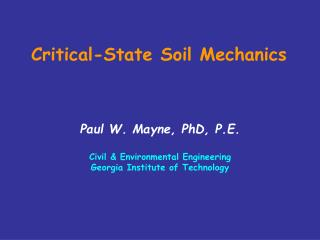DownloadDownload PresentationCritical-State Soil Mechanics

# Critical-State Soil Mechanics

Download Presentation## Critical-State Soil Mechanics

- - - - - - - - - - - - - - - - - - - - - - - - - - - E N D - - - - - - - - - - - - - - - - - - - - - - - - - - -
##### Presentation Transcript

1. Critical-State Soil Mechanics Paul W. Mayne, PhD, P.E.Civil & Environmental EngineeringGeorgia Institute of Technology

2. PROLOGUE • Critical-state soil mechanics is an effective stress framework describing mechanical soil response • In its simplest form here, we consider only shear-induced loading. • We merely tie together two well-known concepts: (1) one-dimensional consolidation behavior, represented by e-logsv’ curves; and (2) shear stress-vs. normal stress (t-sv’) from direct shear box or simple shearing. • Herein, only the bare essence of CSSM concepts are presented, sufficient to describe strength & compressibility response.

3. Critical State Soil Mechanics (CSSM) • Experimental evidence provided by Hvorslev (1936; 1960, ASCE); Henkel (1960, ASCE Boulder) Henkel & Sowa (1961, ASTM STP 361) • Mathematics presented elsewhere, including: Schofield & Wroth (1968); Burland (1968); Wood (1990). • In basic form: 3 material constants (f', Cc, Cs) plus initial state (e0, svo', OCR) • Constitutive Models, include: Original Cam-Clay, Modified Cam Clay, NorSand, Bounding Surface, MIT-E3 (Whittle, 1993) & MIT-S1 (Pestana) and others (Adachi, Oka, Ohta, Dafalias) • "Undrained" is just one specific stress path • Yet !!! CSSM is missing from most textbooks and undergrad & grad curricula in the USA.

4. svo'=300 kPa sp'=900 kPa Cr = 0.04 Cc = 0.38 One-Dimensional Consolidation Overconsolidation Ratio, OCR = 3 Cs = swelling index (= Cr) cv = coef. of consolidation D' = constrained modulus Cae = coef. secondary compression k ≈ hydraulic conductivity sv’

5. sv’ t t t t d gs Direct Simple Shear (DSS) Direct Shear Test Results sv’ Direct Shear Box (DSB)

6. Void Ratio, e NC CSL CSL Effective stress sv' CSL tanf' CSSM CC Void Ratio, e NC Log sv' CSSM Premise: “All stress paths fail on the critical state line (CSL)” Shear stress t f c=0 Effective stress sv'

7. De ef CSL CSSM CC Void Ratio, e Void Ratio, e e0 NC NC CSL CSL svo Effective stress sv' Log sv' tmax = c + s tanf tanf' STRESS PATH No.1 NC Drained Soil Shear stress t Given: e0, svo’, NC (OCR=1) Drained Path: Du = 0 Volume Change is Contractive:evol = De/(1+e0) < 0 c’=0 svo Effective stress sv'

8. e0 svf svo CSL svf svo CSSM CC Void Ratio, e Void Ratio, e NC NC CSL CSL Effective stress sv' Log sv' tanf' STRESS PATH No.2 NC Undrained Soil Du tmax = cu=su Shear stress t Given: e0, svo’, NC (OCR=1) Undrained Path: DV/V0 = 0 +Du = Positive Excess Porewater Pressures Effective stress sv'

9. CSL CSSM CC Void Ratio, e Void Ratio, e NC NC CSL CSL Effective stress sv' Log sv' tanf' Note: All NC undrained stress paths are parallel to each other, thus: su/svo’ = constant Shear stress t DSS: su/svo’NC = ½sinf’ Effective stress sv'

10. CSSM CC Void Ratio, e OC Void Ratio, e CS NC NC CSL CSL Effective stress sv' sp' Log sv' CSL Overconsolidated States: e0, svo’, and OCR = sp’/svo’ where sp’ = svmax’= Pc’ = preconsolidation stress; OCR = overconsolidation ratio tanf' Shear stress t sp' Effective stress sv'

11. e0 svo' svf' Du CSSM CC Void Ratio, e Void Ratio, e OC CS NC NC CSL CSL Effective stress sv' Log sv' CSL Stress Path No. 3 Undrained OC Soil: e0, svo’, and OCR tanf' Shear stress t Stress Path: DV/V0 = 0 Negative Excess Du svo' Effective stress sv'

12. e0 svo' svo' CSSM CC Void Ratio, e Void Ratio, e OC CS NC NC CSL CSL Effective stress sv' Log sv' CSL Stress Path No. 4 Drained OC Soil: e0, svo’, and OCR tanf' Shear stress t Stress Path: Du = 0 Dilatancy: DV/V0 > 0 Effective stress sv'

13. Critical state soil mechanics • Initial state: e0, svo’, and OCR = sp’/svo’ • Soil constants: f’, Cc, and Cs (L = 1-Cs/Cc) • For NC soil (OCR =1): • Undrained (evol = 0): +Du and tmax = su = cu • Drained (Du = 0) and contractive (decreaseevol) • For OC soil: • Undrained (evol = 0): -Du and tmax = su = cu • Drained (Du = 0) and dilative (Increaseevol) There’s more ! Semi-drained, Partly undrained, Cyclic…..

14. e0 De ep svo' se' svf' Equivalent Stress Concept CC NC Void Ratio, e Void Ratio, e NC OC CS sp' sp' CSL CSL Effective stress sv' Log sv' CSL 1. OC State (eo, svo’, sp’) tanf' 2. Project OC state to NC line for equivalent stress, se’ Shear stress t su at se’ suOC = suNC De = Cs log(sp’/svo’) De = Cc log(se’/sp’) 3. se’ = svo’ OCR[1-Cs/Cc] svo' se' Stress sv'

15. Critical state soil mechanics • Previously: su/svo’ = constant for NC soil • On the virgin compression line: svo’ = se’ • Thus: su/se’ = constant for all soil (NC & OC) • For simple shear: su/se’ = ½sin f’ • Equivalent stress: se’ = svo’ OCR[1-Cs/Cc] Normalized Undrained Shear Strength: su/svo’ = ½ sinf’ OCRL where L = (1-Cs/Cc)

16. Undrained Shear Strength from CSSM

17. Undrained Shear Strength from CSSM

18. Porewater Pressure Response from CSSM

19. Yield Surface Yield Surfaces NC NC CSL OC OC Void Ratio, e Void Ratio, e sp' CSL sp' Normal stress sv' Log sv' CSL • Yield surface represents 3-d preconsolidation • Quasi-elastic behavior within the yield surface Shear stress t Normal stress sv'